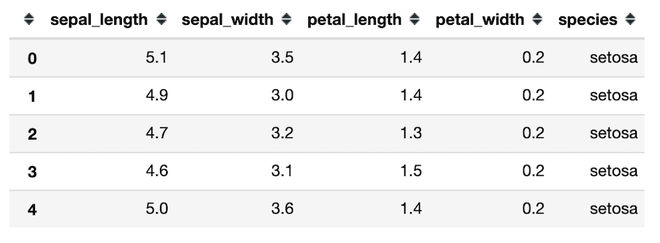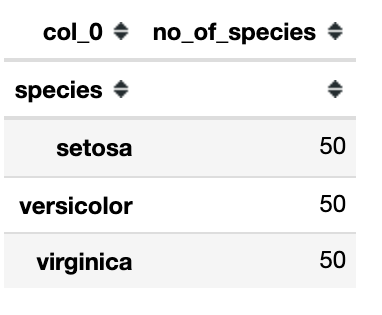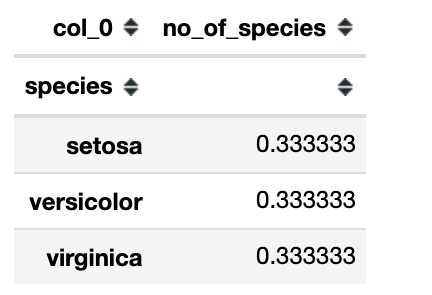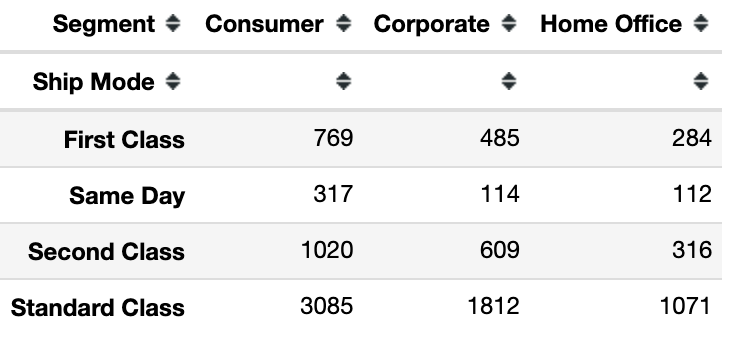Open in App
Not now

# How to Create Frequency Tables in Python?

• Last Updated : 20 Feb, 2022

In this article, we are going to see how to Create Frequency Tables in Python

Frequency is a count of the number of occurrences a particular value occurs or appears in our data. A frequency table displays a set of values along with the frequency with which they appear. They allow us to better understand which data values are common and which are uncommon. These tables are a great method to arrange your data and communicate the results to others. In this article let’s demonstrate the different ways in which we can create frequency tables in python.

## Method 1: Simple frequency table using value_counts() method

Let’s take a look at the dataset we’ll work on :

The necessary packages are imported and the dataset is read using the pandas.read_csv() method. df.head() method returns the first 5 rows of the dataset.

## Python3

 `# import packages ` `import` `pandas as pd ` `import` `numpy as np ` ` `  `# reading csv file as pandas dataframe ` `data ``=` `pd.read_csv(``'iris.csv'``) ` `data.head()`

Output:Now let’s find the one-way frequency table of the species column of the dataset.

## Python3

 `df ``=` `data[``'species'``].value_counts() ` `print``(df) `

Output:

```setosa        50
virginica     50
versicolor    50
Name: species, dtype: int64```

## Method 2: One-way frequency table using pandas.crosstab() method

Here we are going to use crosstab() method to get the frequency.

Syntax: pandas.crosstab(index, columns, values=None, rownames=None, colnames=None, aggfunc=None, margins=False, margins_name=’All’, dropna=True, normalize=False)

Parameters:

• index: array or series which contain values to group by in the rows.
• columns: array or series which contain values to group by in the columns.it’s name we give to the column we find frequency
• values : An array of numbers that will be aggregated based on the factors.

In the below code we use the crosstab function where we give the species column as an index and ‘no_of_species’ as the name of the frequency column.

## Python3

 `# import packages ` `import` `pandas as pd ` `import` `numpy as np ` ` `  `import` `matplotlib.pyplot as plt ` `%``matplotlib inline ` ` `  `# reading csv file as pandas dataframe ` `data ``=` `pd.read_csv(``'iris.csv'``) ` ` `  `# one way frequency table for the species column. ` `freq_table ``=` `pd.crosstab(data[``'species'``], ``'no_of_species'``) ` ` `  `freq_table `

Output: 50 plants belonging to the setosa species, 50 of Versicolor and 50 of Virginica.If we want the frequency table to be in proportions then we’ve to divide each individual proportion by the sum of the total number.

## Python3

 `# import packages ` `import` `pandas as pd ` `import` `numpy as np ` ` `  `import` `matplotlib.pyplot as plt ` `%``matplotlib inline ` ` `  `# reading csv file as pandas dataframe ` `data ``=` `pd.read_csv(``'iris.csv'``) ` ` `  `# one way frequency table for the species column. ` `freq_table ``=` `pd.crosstab(data[``'species'``], ``'no_of_species'``) ` ` `  `# frequency table in proportion of species ` `freq_table``=` `freq_table``/``len``(data) ` ` `  `freq_table `

Output: 0.333 indicates 0.333% of the total population is setosa and so on.## Method 3: Two-way frequency table using pandas.crosstab() method

Two – way frequency table is where we create a frequency table for two different features in our dataset. To download and review the CSV file used in this example click here. In the below example we create a two-way frequency table for the ship mode and segment columns of our dataset.

## Python3

 `# import packages ` `import` `pandas as pd ` `import` `numpy as np ` ` `  `# reading csv file  ` `data ``=` `pd.read_csv(``'SampleSuperstore.csv'``) ` ` `  `# two way frequency table for the ship mode column ` `# and consumer column of the superstore dataset. ` `freq_table ``=` `pd.crosstab(data[``'Ship Mode'``], data[``'Segment'``]) ` ` `  `freq_table`

Output:We can interpret this table as for ship mode first class there are 769 consumer segments, 485 corporate segments and 284 home office segments, and so on.

My Personal Notes arrow_drop_up
Related Articles